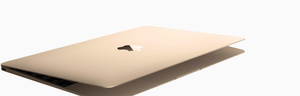# Refurbished Mac

Discover what goes into each refurbished Mac.

A\$1,359.00

A\$1,609.00

A\$1,609.00

A\$1,699.00

A\$1,699.00

A\$1,699.00

A\$1,799.00

A\$1,799.00

A\$1,829.00

A\$1,869.00

A\$1,869.00

A\$1,949.00

A\$1,949.00

A\$1,949.00

A\$1,949.00

A\$1,949.00

A\$1,949.00

A\$2,079.00

A\$2,079.00

A\$2,139.00

A\$2,209.00

A\$2,269.00

A\$2,269.00

A\$2,289.00

A\$2,339.00

A\$2,339.00

A\$2,399.00

A\$2,399.00

A\$2,539.00

A\$2,549.00

A\$2,549.00

A\$2,649.00

A\$2,649.00

A\$2,669.00

A\$2,679.00

A\$2,719.00

A\$2,719.00

A\$2,799.00

A\$2,799.00

A\$2,919.00

A\$2,919.00

A\$2,939.00

A\$2,949.00

A\$3,079.00

A\$3,229.00

A\$3,229.00

A\$3,329.00

A\$3,329.00

A\$3,379.00

A\$3,429.00

A\$3,449.00

A\$3,449.00

A\$3,739.00

A\$3,739.00

A\$3,839.00

A\$3,839.00

A\$3,889.00

A\$3,929.00

A\$3,969.00

A\$3,989.00

A\$3,989.00

A\$4,049.00

A\$4,119.00

A\$4,279.00

A\$4,309.00

A\$4,319.00

A\$4,319.00

A\$4,419.00

A\$4,449.00

A\$4,469.00

A\$4,649.00

A\$4,669.00

A\$4,689.00

A\$4,739.00

A\$4,739.00

A\$4,779.00

A\$4,779.00

A\$4,809.00

A\$4,829.00

A\$4,829.00

A\$4,839.00

A\$4,849.00

A\$4,849.00

A\$4,859.00

A\$4,919.00

A\$4,959.00

A\$4,959.00

A\$4,959.00

A\$4,989.00

A\$5,059.00

A\$5,079.00

A\$5,079.00

A\$5,149.00

A\$5,209.00

A\$5,209.00

A\$5,289.00

A\$5,329.00

A\$5,509.00

A\$5,509.00

A\$5,529.00

A\$5,639.00

A\$5,669.00

A\$5,759.00

A\$6,139.00

A\$6,319.00

A\$6,349.00

A\$6,439.00

A\$6,489.00

A\$6,569.00

A\$7,409.00

A\$7,519.00

A\$8,199.00